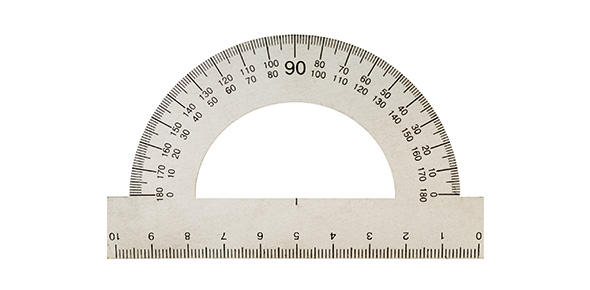# Maps And Measurements Pre-assessment (Earth Science Regents)

10 Questions | Total Attempts: 124SettingsCreate your own QuizMaps and Measurements Pre-Assessment (Earth Science Regents)

• 1.
The diagram below represents part of Earth’s latitude-longitude system. What is the latitude and longitude of point A? (Standard 1.1c)
• A.

15°E , 30°N

• B.

15°S, 30°E

• C.

15°W, 30°S

• D.

15°N, 30°W

• E.

15°S, 30°W

• 2.
Which reference line passes through both the geographic North Pole and the geographic South Pole? (Standard 1.1c).
• A.

0° latitude

• B.

0° longitude

• C.

Tropic of Cancer (23.5°N)

• D.

Tropic of Capricorn (23.5°S)

• E.

No reference line passes through both poles.

• 3.
When the time of day for a certain ship at sea is 12 noon, the time of day at the Prime Meridian (0° longitude) is 5 p.m. What is the ship’s longitude? (Standard 1.1.d)
• A.

75°E

• B.

75°W

• C.

45°E

• D.

45°W

• E.

There is not enough information to answer this question.

• 4.
Base your answer to this question on the topographic map below. Points X, Y, and Z are locations on the map. Elevations are expressed in meters.Which profile best represents the topography along the dashed line from point X to point Y? (Standard 2.1q)a. b.c.d.
• A.

A

• B.

B

• C.

C

• D.

D

• 5.
A stream has a source at an elevation of 1000 meters. It ends in a lake that has an elevation of 300 meters. If the lake is 200 kilometers away from the source, what is the average gradient of the stream? (Standard 2.1q) Gradient = Change in Field Value ÷ Change in Distance
• A.

1.5 m/km

• B.

3.5 m/km

• C.

10 m/km

• D.

15 m/km

• E.

1500 m/km

• 6.
Base your answer to this question on the topographic map below. Points X, Y, and Z are locations on the map. Elevations are expressed in meters.Mill River generally flows toward the (Standard 2.1q):
• A.

Southeast

• B.

Southwest

• C.

Northeast

• D.

Northwest

• E.

True west

• 7.
Base your answer to this question on the topographic map below. Points X, Y, and Z are locatons on the map. Elevations are expressed in meters.What is the elevation of point Z? (Standard 2.1q)?
• A.

190 m

• B.

220 m

• C.

240 m

• D.

250 m

• E.

300 m

• 8.
Base your answer to this question on the map below, which shows most of New York State. Isolines indicate the depth of the Precambrian bedrock surface below present-day sea level. Depths are in feet.Which map best shows the location of the -4,000-foot isoline? a. b.c.d.
• A.

A

• B.

B

• C.

C

• D.

D

• 9.
Rate, on a scale of 1 – 4, 1 being the lowest and 4 being the highest, how much you already know about maps and measurement.
• 10.
Rate, on a scale of 1 – 4, 1 being the lowest and 4 being the highest, how interested are you in maps and measurement
Related TopicsBack to top
×

Wait!
Here's an interesting quiz for you.